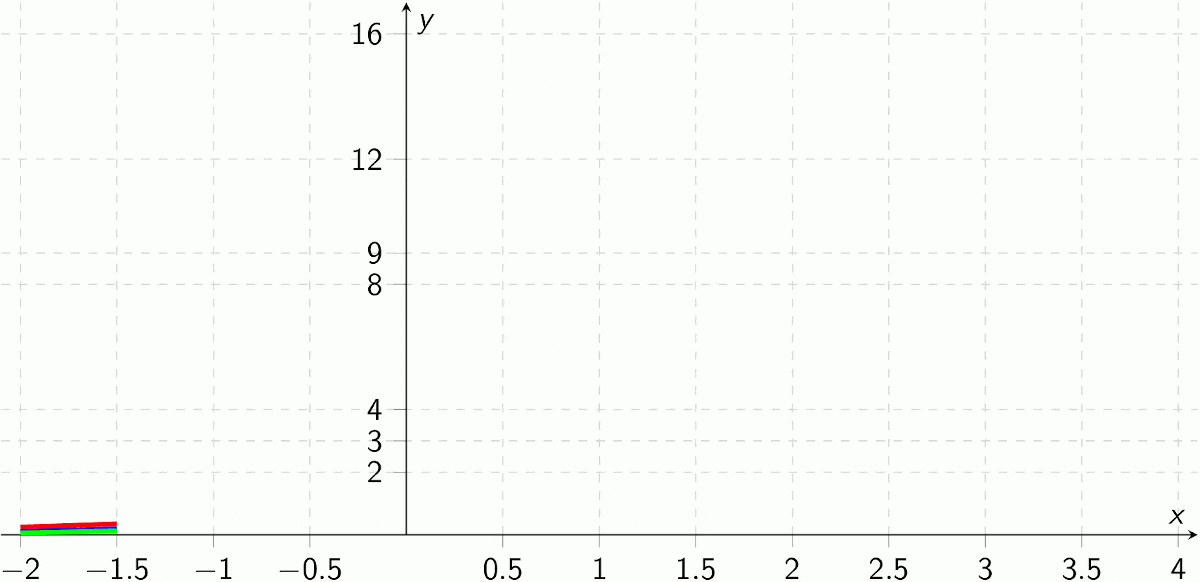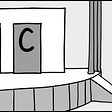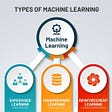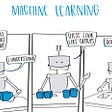# Week 1: Data Science Math Skills

What is Set?

Cardinality

Intersections

Unions

Medical Testing Example (test and cardinality)

Venn Diagrams

Inclusion-Exclusion Formula

# Intersections

`A ∩ C = ∅ (∅, is known as the empty set)`

# Medical Testing Example

`S ∩ H = ∅ (No one person is both S and H)S ∪ H = X ( either person have VBS or not)`
`P ∩ N = ∅ (No one tests is both P and N)P ∪ N = X (Either test is P or N)`
`|S|───  = proportion of people who have VBS|X||H|───  = proportion of people who do not have VBS|X||S∩P|─────  = true positive rate  |X||H∩N|─────  = true negative rate  |X||H∩P|─────  = false positive rate  |X||S∩N|─────  = false negative rate  |X|`

# Inclusion-Exclusion Formula

`|A ⋃ B| = |A| + |B|- |A ∩ B|`
`|A ⋃ B| = 8, {1,9,6,3,4,8} |A| = 5, {1,9,6,5,7}|B| = 5, {3,4,8,5,7}|A ∩ B| = 2, {5,7}|A ⋃ B| = |A| + |B|- |A ∩ B|8 = 5 + 5–28 = 10 -28 = 8`

Real Numbers : Integers and rational numbers

Absolute value

Intervals and Interval Notation

# Absolute Value

`|x| = x, if x is non-negative|x| = -x, if x is negative`

# Inequalities

`a < b “ a is less than b”a > b “a is greater than b”a ≤ b “a is less than or equal to b”a ≥ b “a is greater than or equal to b”a << b “a is much much lesser than b”`

# Half-open intervals

Sigma Notation

Distributive and Commutative property

Summation of constants

Mean and Variance

# Sigma Notation

`i is just an “dummy indices”, that is used as a counter.`

# Mean and Variance

--

--

--

Programming , Ethical Hacking and Cyber Security

Love podcasts or audiobooks? Learn on the go with our new app.

## Qubit, An Intuition #2 — Inner Product, Outer Product, and Tensor Product in Bra-ket Notation## The 5 functions you need to know## Which books are best for the preparation of the JEE Mains and Advanced?## DP Binomial Coefficient(MondayAlgo #7)## The Fibonacci Formula## The Monty Hall Problem and The Bayes’ Theorem## Nishant Tiwari

Programming , Ethical Hacking and Cyber Security

## Machine Learning## Machine Learning in Action — Supervised Learning## All about statistics for Data Science(part1)